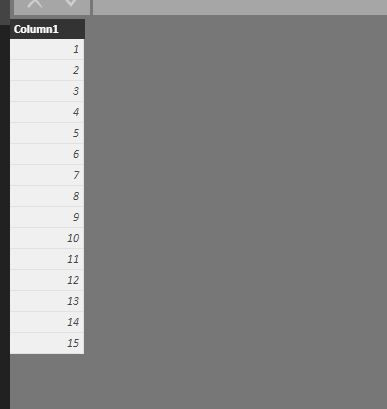cancel
Showing results for
Did you mean:Helper III

## Multiplying a column based on slicer

Hi,

I have a data model where I have a day in the year and a certain utlization in one table. For example Day 1 = 0.4, Day 2 = 0.6. Now I also have a table which lists values between 1 and 15. I have put this table in a slicer with 15 different options.

Is it possible to now multiply my utilization by the number selected in my slicer i.e.:

Slicer selection = 4

Day 1 = 1.6

Day 2 = 3.2

I'm not sure if this is possible or not?

Kind regards,

Matthias

2 ACCEPTED SOLUTIONSMicrosoft

@Matthias93,

The value of Day 2 should be 2.4(4*0.6), right? If so, I make a test in the following sample tables.Create the following measure in the first table.

`multiplyMeasure = MAX(Table2[Column1])*MAX(Table1[Column1])`

Create a table visual and  test the slicer.Regards,
Lydia

Community Support Team _ Lydia Zhang
If this post helps, then please consider Accept it as the solution to help the other members find it more quickly.Helper III

Hi Lydia,

This is exactly what I needed for my dashboard. Thanks for your quick help, I'll try to apply it to my case now.

Kind regards,

Matthias

6 REPLIES 6Microsoft

@Matthias93,

The value of Day 2 should be 2.4(4*0.6), right? If so, I make a test in the following sample tables.Create the following measure in the first table.

`multiplyMeasure = MAX(Table2[Column1])*MAX(Table1[Column1])`

Create a table visual and  test the slicer.Regards,
Lydia

Community Support Team _ Lydia Zhang
If this post helps, then please consider Accept it as the solution to help the other members find it more quickly.Regular Visitor

Hello Lydia!

Your solution worked great, but I was wondering if your solution also applies when using 2 slicers. What I mean is to first choose an option of multiplier, and then choosing a second option to again multiply the total amount from the previous operation.

Thanks!

JuanHelper III

Hi Lydia,

This is exactly what I needed for my dashboard. Thanks for your quick help, I'll try to apply it to my case now.

Kind regards,

MatthiasMicrosoft

@Matthias93,

Regards,
Lydia

Community Support Team _ Lydia Zhang
If this post helps, then please consider Accept it as the solution to help the other members find it more quickly.Helper III

Hi,

I have a data model where I have a day in the year and a certain utlization in one table. For example Day 1 = 0.4, Day 2 = 0.6. Now I also have a table which lists values between 1 and 15. I have put this table in a slicer with 15 different options.

Is it possible to now multiply my utilization by the number selected in my slicer i.e.:

Slicer selection = 4

Day 1 = 1.6

Day 2 = 3.2

I'm not sure if this is possible or not?

Kind regards,

MatthiasCommunity Champion

@Matthias93

You can use the SELECTEDVALUE function

SelectedVALUE(SlicerTableName[SlicerColumnName]) should give you the value selected in the slicer,,, then you can multiply it with any other MEASURE

However you can not use it in a calculated column

Regards
Zubair